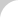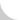The Complete Guide to Chain# 2.3.1 Coefficient of Friction

The tension of transmission chain is calculated by dividing the transmitted power (indicated as kW or horsepower) by the chain speed and multiplying by an adequate coefficient. But in a fixed-speed, horizontal conveyor, tension is decided by those factors shown below:

1. The coefficient of friction between the chain and the rail when conveyed objects are placed on the chain.
2. The coefficient of friction between conveyed objects and the rail when conveyed objects are held on the rail and pushed by the chain.

NOTE: There are two types of tension: the first occurs when conveyed objects are moving at a fixed speed, and the second is inertial effects that occur when starting and stopping the machine. We will only talk about the former in this section, and the latter in Basics Section 2.3.2.Figure 2.22 Tension on a Horizontal Conveyor

The tension (T) in a horizontal conveyor, like that in Figure 2.22, is basically calculated by this formula:

T = M1 × g × f1 × 1.1 + M1 × g × f2 + M2 × g × f3

Where:

• T = total chain tension
• M1 = weight of the chain, etc.
• M2 = weight of conveyed objects
• f1 = coefficient of friction when chain, etc., are returning
• f2 = coefficient of friction when chain, etc., are conveying
• f3 = coefficient of friction when conveyed objects are moving
• g = gravitational constant
• 1.1 = sprocket losses due to directional changes of the chain

NOTE: "chain, etc.," in the above formula includes chain and the parts moving with the chain, such as attachments and slats.

In this formula, a coefficient of friction is multiplied by every term in the equation. Therefore, if the coefficient of friction is high, the tension increases and larger chain is required. Also, the necessary motor power, which is calculated as tension × speed × coefficient, increases. A more powerful motor is needed when the coefficient of friction is high.

Reduce the coefficient of friction and you can reduce the tension, too. This allows you to choose a more economical chain and motor, and decrease the initial and running costs for conveyor equipment.

The chain's coefficient of friction differs by type of chain, by material, and by type of roller; it is shown in the manufacturer's catalog. To illustrate this concept, two examples are included. The coefficient of friction for different types of top chain and guide rails is shown in Table 2.3. The coefficient of friction when large R-roller chain rotates on rails (rail material: steel) is shown in Table 2.4.

Friction Coefficient Top Plate Material Guide Rail Material Unlubricated Stainless Steel or Steel Stainless Steel or Steel 0.35 0.20 Stainless Steel or Steel UHMW 0.25 0.15 Engineered Plastic Stainless Steel or Steel 0.25 0.15 Engineered Plastic UHMW 0.25 0.12 Engineered Plastic (Low Friction) Stainless Steel or Steel 0.17 0.12 Engineered Plastic (Low Friction UHMW 0.18 0.12
Table 2.4 Friction Coefficients for Different Types of Rollers
Friction Coefficient
Chain Type Roller Type Unlubricated Lubricated
RF Double Pitch Chain Steel0.12 0.08
Engineered Plastic0.08
Large Pitch Conveyor Chain Steel0.13~0.15 0.08
Engineered Plastic0.08
Bearing Roller0.03

Technology can help you reduce the coefficient of friction. Some of the newest chains (for example, low-friction top chain, engineered plastic roller chain, and bearing roller chain) can achieve low coefficients of friction without lubrication. Other chains would have to be lubricated to achieve these coefficients. In some instances, these new chains achieve dramatically lower coefficients of friction. That means you can save maintenance time, money, and energy at your facility.